Courses

# Description of General VLE Behavior Civil Engineering (CE) Notes | EduRev

## Civil Engineering (CE) : Description of General VLE Behavior Civil Engineering (CE) Notes | EduRev

The document Description of General VLE Behavior Civil Engineering (CE) Notes | EduRev is a part of the Civil Engineering (CE) Course Thermodynamics.
All you need of Civil Engineering (CE) at this link: Civil Engineering (CE)

Description of General VLE Behavior

Before presenting the mathematical formulation of the multi-component VLE problem it is pertinent to discuss some key features of typical vapour liquid phase behaviour. The description of phase behaviour of vapour and liquid phases co-existing under equilibrium can be complex and difficult to visualize for systems containing a large number of chemical species. Thus, to clarify matters it is useful to consider a binary system. The considerations for such a system may, in principle, be generalized to understand the behaviour of multi-component systems. However, we restrict ourselves to description of VLE of multi-component systems to the corresponding mathematical formulation.   When N = 2, the phase rule yields a degree of freedom F = 4 – π. In the general VLE problem since there are at least two phases, the corresponding number of independent intensive variables become 2. In the case of extractive distillation, there are at least two liquid phases and a vapour phase, whence the degrees of freedom reduce to 1.In the following discussion we present the phase behaviour of the simplest case of a binary VLE. The more volatile of the two is designated as component (1). The phase rule variables for this case are: T, P, y1 and x1 . Graphical plots of experimentally obtained phase behaviour can then be expressed as a functionof various combinations of these variables. For example at a given temperature one may plot two curves, P vs. x1 (or in short P- x1 ) and P vs. y1 . Similarly on specifying a certain pressure, one may plot T vs. x1 and T vs. y1 . In addition if one fixes either x1 or y 1 one may plot P-T diagrams. The combination of these diagrams lead to a 3-dimensional surface involving pressure, temperature and phase compositions on the three axes. A typical plot is shown in fig. 7.2.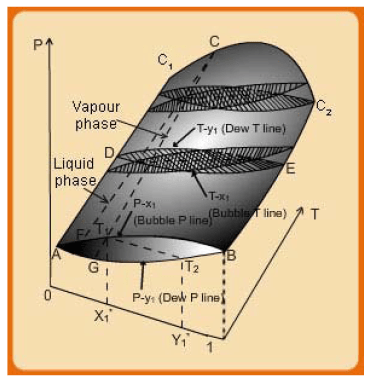Fig. 7.2 Three dimensional VLE phase diagram for a binary system

Consider first a case of VLE phase behaviour at a constant temperature. This is in shown as the “lens” AB. The lower part of the lens corresponds to P-y1  while upper curve is the P-xplot. The values of the vapour and liquid compositions at any system equilibrium pressure are found by drawing a line parallel to the axis. The point of intersection of this line with the upper curve provides the saturated liquid phase composition ( x1 ) and is termed the bubble pressure line; while that with the lower curve corresponds to the saturated vapour phase composition ( y1) and is termed the dew pressure line. Extending the description the entire upper face of the three dimensional surface constitutes the bubble surface. Any point above it corresponds to the state of sub-cooled liquid. In the same manner, the lower face represents the dew surface. For any point below this face the state is that of a superheated vapour. The line that connects the phase compositions ( x1* and y1* ) is called the tie line (shown as T1-T2). Such tie lines may be drawn at any other pressure and the same considerations as above are valid.

Similarly, if one considers isobaric plots (shown as the lens DE), for any equilibrium temperature the vapour and liquid phase compositions are found by drawing a line that passes through the specific temperature and is parallel to the x1−y1 axis. The upper intersection point provides the vapour phase composition (dew temperature line), while the lower one corresponds to the liquid phase composition (bubble temperature line). Finally if one fixes the composition at a point on thex1−yaxis the intersection of a vertical plane through the composition point with the 3-dimension surface yields the curve FCG. The upper part of this curve, i.e., FC corresponds to the locus of liquid phase compositions while the lower one (CG) is that of the vapour phase compositions. The point C is the meeting point of the two curves and defines the critical point of the mixture at the composition specified by the original vertical plane at a point on the x1−y1 axis. At the two end points of the composition axis are the pure component P-T plots which terminate at the critical points,C1-C2, of the two substances.

If one takes a series of varying isothermal or isobaric “lenses”, two types of plots result, which are shown in fig. 7.3. Consider the P-x-y plot. The lowest lens (TX) corresponds to the situation already described in the last paragraph (i.e., the lens MN). However, the one at TY corresponds to a case for which  TC1<TY, but TC2>TY. Thus at this temperature the vapour and liquid phases of the pure component ‘1’ cannot co-exist, and hence the P-x-y plot vanishes as the composition tends to x1 → 1.If one moves to a still higher temperature say TZ  the P-x-y “hangs” at both ends of the x1 −y1 as both Tc1  < Tz and Tc2 <Tz The considerations for the isobaric lenses at PX , PY and PZ are the same as for the isotherms, i,e., for the highest isobar, both  PC1 <PZ , and PC2< PZ and so on.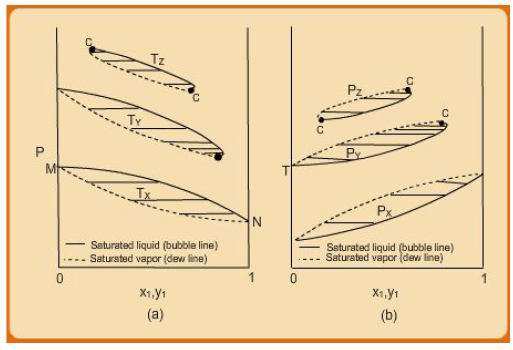Fig. 7.3 (a) P-x1 –y1 diagrams for three temperatures. (b) T-x1 -y1 diagrams for three pressures

If one considers now a series of P-T plots they correspond to the curves shown in fig, 7.4. The lines I-J and K-L which represent the vapour pressure-vs.-T curves for pure species 1 and 2 respectively. At other intermediate compositions the upper and lower plots (obtained by intersection of a vertical plane at constant point on the x1 −y1 axis, and the P-T-x-y surface of fig. 7.2) constitute a curve that rounds off at the true critical point of the mixture (as opposed to the “pseudo-critical” temperature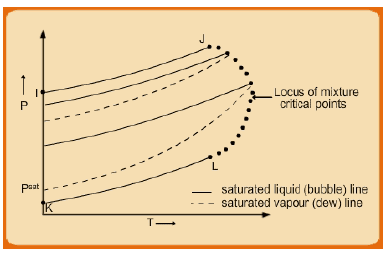Fig. 7.4 P-T diagram for various compositions

and pressure of mixtures discussed in section 2.4).  The critical points of the various mixtures of the two species thus lie along a line on the rounded edge of the surface between J and L; it is, therefore composition dependent. Each interior loop represents the P-T behavior of saturated liquid and of saturated vapour for a mixture of constant composition; the loops differ from one composition to another. It follows that the P-T relation for saturated liquid is different from that for saturated vapour of the same composition. This is in contrast with the behavior of a pure species, for which the bubble and dew lines are the same, as for I-J and K-L.

The above discussion suggests that the phase behaviour of even a simple binary can be relatively more difficult to interpret in its complete three-dimensional form. Reducing such behaviour to two-dimensional plots enables easier visualization of the phase behaviour and understanding their features. However, in many instances, even two dimensional plots can be more complex in nature. Examples of such curves (as are often encountered with solvent mixtures in industrial practice) are shown schematically in the form of (P-x-y) in fig. 7.5, for systems at relatively low pressures (≤ 1 atm).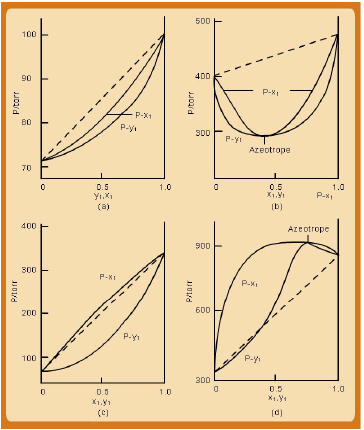Fig. 7.5 Schematic P-x-y plots showing deviation from ideal VLE behaviour

All the four systemic VLE behaviour exhibits deviation from ideal vapour-liquid systems. As discussed in the next section ideal VLE behaviour is characterized by Raoult’s Law (RL). Suffice it mention here that non-ideal VLE systems may exhibit both negative (figs. 7.5a & 7.5b) and positive deviations (figs. 7.5c and 7.5d)  from that which obeys the Raoult’s law (RL). The RL behaviour is typified by the dotted P-x1 lines in each set in fig. 7.5. When the actual P-x1  line lies below the RLline, the system is said to show negative deviation from RL, while if it lies above it is indicative of a positive deviation.

From a molecular thermodynamic viewpoint, negative deviations occur if the 1-2 type (between unlike species) of molecular interactions (attraction) are stronger than 1-1 or 2-2 (between like species) type of interaction. As a result, the molecules of the more volatile component (1) are “constrained” by those of component 2 from transiting to the vapour phase to a greater extent than in the case when the former is present in a pure form. This effectively translates into an equilibrium pressure less than the RL system at the same liquid phase composition; hence the actual system displays “negative” deviation in comparison to RL for which 1-1, 2-2, and 1-2 types of interactions are more or less the same.  Appreciable negative departures fromP-xlinearity reflect strong liquid-phase intermolecular interaction. The opposite applies to the case where a system shows a positive deviation from RL; that is, the interaction between the unlike species of molecules is lower than that between like molecules.

There is an additional complexity evident in figs. 7.5c and 7.5d. Considering the former we see that it is defined by two distinct types of behaviour on either sides of the point termed as the “azeotrope”. At this point the P-xand the P-y  curves converge; i.e., the two phases are identical in composition at this point. On left side of the azeotrope , while on the other side, x <y. The reverse situation holds for the system depicted in fig. 7.4d. Such systems are not uncommon in the process industry and always pose a difficulty in purifying a mixture to compositions higher than the azeotropic point. This is because during a distillation process when the mixture composition arrives at the azeotropic point the two phases become identical in composition, and the liquid composition does not alter further during evaporation.

The phase behaviour of the same systems as in fig. 7.5 is depicted as T-x-y diagrams in fig. 7.6. In particular we refer to the figs 7.6b and 7.6d. As expected the P-x curves appear inverted on a T-x diagram. The first kind of system (fig. 7.6b) is said to show a maximum boiling point azeotrope, while that depicted by fig. 7.5d shows a minimum boiling point azeotropic behaviour.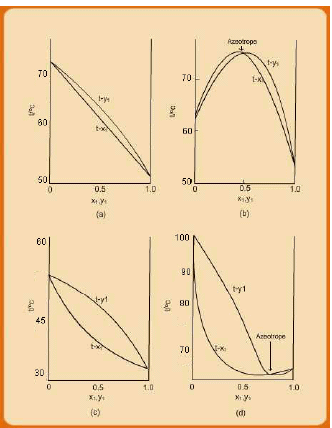Fig. 7.6 Schematic T-x-y plots showing deviation from ideal VLE behaviour

Offer running on EduRev: Apply code STAYHOME200 to get INR 200 off on our premium plan EduRev Infinity!

## Thermodynamics

56 videos|92 docs|33 tests

,

,

,

,

,

,

,

,

,

,

,

,

,

,

,

,

,

,

,

,

,

;